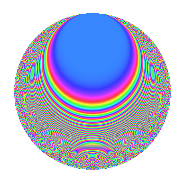# Properties

 Label 1342.2.aLevel 1342 Weight 2 Character orbit a Rep. character $$\chi_{1342}(1,\cdot)$$ Character field $$\Q$$ Dimension 49 Newforms 14 Sturm bound 372 Trace bound 5

# Related objects

## Defining parameters

 Level: $$N$$ = $$1342 = 2 \cdot 11 \cdot 61$$ Weight: $$k$$ = $$2$$ Character orbit: $$[\chi]$$ = 1342.a (trivial) Character field: $$\Q$$ Newforms: $$14$$ Sturm bound: $$372$$ Trace bound: $$5$$ Distinguishing $$T_p$$: $$3$$, $$5$$

## Dimensions

The following table gives the dimensions of various subspaces of $$M_{2}(\Gamma_0(1342))$$.

Total New Old
Modular forms 190 49 141
Cusp forms 183 49 134
Eisenstein series 7 0 7

The following table gives the dimensions of the cuspidal new subspaces with specified eigenvalues for the Atkin-Lehner operators and the Fricke involution.

$$2$$$$11$$$$61$$FrickeDim.
$$+$$$$+$$$$+$$$$+$$$$6$$
$$+$$$$+$$$$-$$$$-$$$$6$$
$$+$$$$-$$$$+$$$$-$$$$9$$
$$+$$$$-$$$$-$$$$+$$$$4$$
$$-$$$$+$$$$+$$$$-$$$$6$$
$$-$$$$+$$$$-$$$$+$$$$6$$
$$-$$$$-$$$$+$$$$+$$$$4$$
$$-$$$$-$$$$-$$$$-$$$$8$$
Plus space$$+$$$$20$$
Minus space$$-$$$$29$$

## Trace form

 $$49q$$ $$\mathstrut -\mathstrut q^{2}$$ $$\mathstrut +\mathstrut 49q^{4}$$ $$\mathstrut -\mathstrut 2q^{5}$$ $$\mathstrut -\mathstrut 4q^{6}$$ $$\mathstrut -\mathstrut 8q^{7}$$ $$\mathstrut -\mathstrut q^{8}$$ $$\mathstrut +\mathstrut 41q^{9}$$ $$\mathstrut +\mathstrut O(q^{10})$$ $$49q$$ $$\mathstrut -\mathstrut q^{2}$$ $$\mathstrut +\mathstrut 49q^{4}$$ $$\mathstrut -\mathstrut 2q^{5}$$ $$\mathstrut -\mathstrut 4q^{6}$$ $$\mathstrut -\mathstrut 8q^{7}$$ $$\mathstrut -\mathstrut q^{8}$$ $$\mathstrut +\mathstrut 41q^{9}$$ $$\mathstrut -\mathstrut 6q^{10}$$ $$\mathstrut +\mathstrut q^{11}$$ $$\mathstrut -\mathstrut 14q^{13}$$ $$\mathstrut -\mathstrut 8q^{14}$$ $$\mathstrut +\mathstrut 8q^{15}$$ $$\mathstrut +\mathstrut 49q^{16}$$ $$\mathstrut +\mathstrut 14q^{17}$$ $$\mathstrut -\mathstrut 13q^{18}$$ $$\mathstrut -\mathstrut 12q^{19}$$ $$\mathstrut -\mathstrut 2q^{20}$$ $$\mathstrut -\mathstrut 8q^{21}$$ $$\mathstrut -\mathstrut q^{22}$$ $$\mathstrut +\mathstrut 4q^{23}$$ $$\mathstrut -\mathstrut 4q^{24}$$ $$\mathstrut +\mathstrut 47q^{25}$$ $$\mathstrut -\mathstrut 2q^{26}$$ $$\mathstrut +\mathstrut 24q^{27}$$ $$\mathstrut -\mathstrut 8q^{28}$$ $$\mathstrut +\mathstrut 10q^{29}$$ $$\mathstrut -\mathstrut 4q^{31}$$ $$\mathstrut -\mathstrut q^{32}$$ $$\mathstrut +\mathstrut 4q^{33}$$ $$\mathstrut -\mathstrut 10q^{34}$$ $$\mathstrut +\mathstrut 32q^{35}$$ $$\mathstrut +\mathstrut 41q^{36}$$ $$\mathstrut -\mathstrut 6q^{37}$$ $$\mathstrut -\mathstrut 6q^{40}$$ $$\mathstrut +\mathstrut 14q^{41}$$ $$\mathstrut +\mathstrut 24q^{42}$$ $$\mathstrut -\mathstrut 28q^{43}$$ $$\mathstrut +\mathstrut q^{44}$$ $$\mathstrut -\mathstrut 2q^{45}$$ $$\mathstrut -\mathstrut 16q^{46}$$ $$\mathstrut -\mathstrut 12q^{47}$$ $$\mathstrut +\mathstrut 17q^{49}$$ $$\mathstrut -\mathstrut 31q^{50}$$ $$\mathstrut -\mathstrut 16q^{51}$$ $$\mathstrut -\mathstrut 14q^{52}$$ $$\mathstrut -\mathstrut 14q^{53}$$ $$\mathstrut +\mathstrut 8q^{54}$$ $$\mathstrut +\mathstrut 6q^{55}$$ $$\mathstrut -\mathstrut 8q^{56}$$ $$\mathstrut -\mathstrut 32q^{57}$$ $$\mathstrut -\mathstrut 22q^{58}$$ $$\mathstrut +\mathstrut 12q^{59}$$ $$\mathstrut +\mathstrut 8q^{60}$$ $$\mathstrut -\mathstrut q^{61}$$ $$\mathstrut +\mathstrut 24q^{62}$$ $$\mathstrut +\mathstrut 49q^{64}$$ $$\mathstrut -\mathstrut 12q^{65}$$ $$\mathstrut -\mathstrut 4q^{66}$$ $$\mathstrut -\mathstrut 36q^{67}$$ $$\mathstrut +\mathstrut 14q^{68}$$ $$\mathstrut +\mathstrut 80q^{69}$$ $$\mathstrut -\mathstrut 16q^{70}$$ $$\mathstrut -\mathstrut 4q^{71}$$ $$\mathstrut -\mathstrut 13q^{72}$$ $$\mathstrut -\mathstrut 10q^{73}$$ $$\mathstrut -\mathstrut 14q^{74}$$ $$\mathstrut +\mathstrut 8q^{75}$$ $$\mathstrut -\mathstrut 12q^{76}$$ $$\mathstrut -\mathstrut 8q^{77}$$ $$\mathstrut +\mathstrut 8q^{78}$$ $$\mathstrut -\mathstrut 32q^{79}$$ $$\mathstrut -\mathstrut 2q^{80}$$ $$\mathstrut +\mathstrut 25q^{81}$$ $$\mathstrut -\mathstrut 10q^{82}$$ $$\mathstrut +\mathstrut 52q^{83}$$ $$\mathstrut -\mathstrut 8q^{84}$$ $$\mathstrut -\mathstrut 36q^{85}$$ $$\mathstrut -\mathstrut 20q^{86}$$ $$\mathstrut -\mathstrut 8q^{87}$$ $$\mathstrut -\mathstrut q^{88}$$ $$\mathstrut +\mathstrut 42q^{89}$$ $$\mathstrut -\mathstrut 6q^{90}$$ $$\mathstrut -\mathstrut 56q^{91}$$ $$\mathstrut +\mathstrut 4q^{92}$$ $$\mathstrut +\mathstrut 24q^{93}$$ $$\mathstrut +\mathstrut 16q^{94}$$ $$\mathstrut +\mathstrut 24q^{95}$$ $$\mathstrut -\mathstrut 4q^{96}$$ $$\mathstrut -\mathstrut 46q^{97}$$ $$\mathstrut +\mathstrut 7q^{98}$$ $$\mathstrut +\mathstrut 13q^{99}$$ $$\mathstrut +\mathstrut O(q^{100})$$

## Decomposition of $$S_{2}^{\mathrm{new}}(\Gamma_0(1342))$$ into irreducible Hecke orbits

Label Dim. $$A$$ Field CM Traces A-L signs $q$-expansion
$$a_2$$ $$a_3$$ $$a_5$$ $$a_7$$ 2 11 61
1342.2.a.a $$1$$ $$10.716$$ $$\Q$$ None $$-1$$ $$1$$ $$-2$$ $$4$$ $$+$$ $$+$$ $$+$$ $$q-q^{2}+q^{3}+q^{4}-2q^{5}-q^{6}+4q^{7}+\cdots$$
1342.2.a.b $$1$$ $$10.716$$ $$\Q$$ None $$1$$ $$-1$$ $$-4$$ $$-2$$ $$-$$ $$-$$ $$-$$ $$q+q^{2}-q^{3}+q^{4}-4q^{5}-q^{6}-2q^{7}+\cdots$$
1342.2.a.c $$1$$ $$10.716$$ $$\Q$$ None $$1$$ $$-1$$ $$0$$ $$-2$$ $$-$$ $$-$$ $$+$$ $$q+q^{2}-q^{3}+q^{4}-q^{6}-2q^{7}+q^{8}+\cdots$$
1342.2.a.d $$1$$ $$10.716$$ $$\Q$$ None $$1$$ $$3$$ $$0$$ $$2$$ $$-$$ $$-$$ $$-$$ $$q+q^{2}+3q^{3}+q^{4}+3q^{6}+2q^{7}+q^{8}+\cdots$$
1342.2.a.e $$2$$ $$10.716$$ $$\Q(\sqrt{2})$$ None $$-2$$ $$2$$ $$0$$ $$0$$ $$+$$ $$+$$ $$-$$ $$q-q^{2}+(1+\beta )q^{3}+q^{4}+\beta q^{5}+(-1+\cdots)q^{6}+\cdots$$
1342.2.a.f $$2$$ $$10.716$$ $$\Q(\sqrt{5})$$ None $$2$$ $$3$$ $$2$$ $$1$$ $$-$$ $$-$$ $$-$$ $$q+q^{2}+(1+\beta )q^{3}+q^{4}+2\beta q^{5}+(1+\cdots)q^{6}+\cdots$$
1342.2.a.g $$3$$ $$10.716$$ 3.3.148.1 None $$3$$ $$-3$$ $$-4$$ $$-4$$ $$-$$ $$-$$ $$+$$ $$q+q^{2}+(-1-\beta _{2})q^{3}+q^{4}+(-1-\beta _{1}+\cdots)q^{5}+\cdots$$
1342.2.a.h $$4$$ $$10.716$$ 4.4.14656.1 None $$-4$$ $$-2$$ $$-2$$ $$-2$$ $$+$$ $$-$$ $$-$$ $$q-q^{2}+(-1+\beta _{1})q^{3}+q^{4}+(-1-\beta _{2}+\cdots)q^{5}+\cdots$$
1342.2.a.i $$4$$ $$10.716$$ 4.4.48396.1 None $$-4$$ $$-2$$ $$0$$ $$3$$ $$+$$ $$+$$ $$-$$ $$q-q^{2}+(-1+\beta _{1})q^{3}+q^{4}+(-\beta _{2}+\cdots)q^{5}+\cdots$$
1342.2.a.j $$4$$ $$10.716$$ 4.4.2225.1 None $$4$$ $$-2$$ $$4$$ $$1$$ $$-$$ $$-$$ $$-$$ $$q+q^{2}+(-1-\beta _{2})q^{3}+q^{4}+(1+\beta _{3})q^{5}+\cdots$$
1342.2.a.k $$5$$ $$10.716$$ 5.5.3362852.1 None $$-5$$ $$-2$$ $$0$$ $$-3$$ $$+$$ $$+$$ $$+$$ $$q-q^{2}-\beta _{1}q^{3}+q^{4}+\beta _{3}q^{5}+\beta _{1}q^{6}+\cdots$$
1342.2.a.l $$6$$ $$10.716$$ 6.6.8248384.1 None $$6$$ $$-3$$ $$-4$$ $$-9$$ $$-$$ $$+$$ $$-$$ $$q+q^{2}+(-1-\beta _{3})q^{3}+q^{4}+(\beta _{3}-\beta _{5})q^{5}+\cdots$$
1342.2.a.m $$6$$ $$10.716$$ 6.6.136632976.1 None $$6$$ $$2$$ $$2$$ $$5$$ $$-$$ $$+$$ $$+$$ $$q+q^{2}+\beta _{1}q^{3}+q^{4}-\beta _{2}q^{5}+\beta _{1}q^{6}+\cdots$$
1342.2.a.n $$9$$ $$10.716$$ $$\mathbb{Q}[x]/(x^{9} - \cdots)$$ None $$-9$$ $$5$$ $$6$$ $$-2$$ $$+$$ $$-$$ $$+$$ $$q-q^{2}+(1-\beta _{1})q^{3}+q^{4}+(1-\beta _{5})q^{5}+\cdots$$

## Decomposition of $$S_{2}^{\mathrm{old}}(\Gamma_0(1342))$$ into lower level spaces

$$S_{2}^{\mathrm{old}}(\Gamma_0(1342)) \cong$$ $$S_{2}^{\mathrm{new}}(\Gamma_0(11))$$$$^{\oplus 4}$$$$\oplus$$$$S_{2}^{\mathrm{new}}(\Gamma_0(61))$$$$^{\oplus 4}$$$$\oplus$$$$S_{2}^{\mathrm{new}}(\Gamma_0(122))$$$$^{\oplus 2}$$$$\oplus$$$$S_{2}^{\mathrm{new}}(\Gamma_0(671))$$$$^{\oplus 2}$$